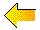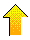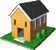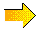# Narcissistic Numbers

Narcissus, according to Greek mythology, fell in love with his own image,
seen in a pool of water, and changed into the flower now called by his name.
Since this section deals with numbers "in love with themselves",  narcissistic
numbers
will be defined as those that are representable, in some way, by
mathematically manipulating the digits of the numbers themselves.

Definition by Joseph S. Madachy, Mathematics on Vacation, Thomas Nelson & Sons Ltd. 1966
He has lots of material on narcissistic numbers on p 163 to 175 of this book.

### Noteworthy Numbers

Ascending & Descending Ascending Powers

Amicable pairs

### PPDI (Armstrong) Numbers

Recurring Digital Invariant Example strings to  PPDI

### Happy Number 7

Summary: PDI, PPDI, RDI, Summary Table of PPDI's,### Constant Base numbers

 4624 = 44+46+42+441033 = 81+80+83+83 Note that the powers match the digits of the number. 595968 = 45+49+45+49+46+48 3909511 = 53+59+50+59+55+51+51 13177388 = 71+73+71+77+77+73+78+78 52135640 = 195+192+191+193+195+196+194+190 To the left are some of those sent to me by Patrick de Geest in Dec.,1998, along with the name suggestion.3435 = 33 + 44 + 33 +55

438579088 = 44 + 33 + 88 +55 + 77 + 99 + 00 + 88 + 88

These are called Perfect Digit-to-Digit Invariants or PDDI's for short. (See PDI, PPDI and RDI at the bottom of this page.)
The only two integers in the decimal number system with this property (plus the trivial 0 & 1).
D. Morrow ran a search up to 109 with no additional finds accept the trivial adding of zeros to the above 2 numbers.
Note that here 00 is considered equal to 0. Normally 00 is considered equal to 1  (see above 1033 where 80 = 1).
Curious and Interesting Numbers p.190 and D. Morrow JRM 27:1, 1995 p 9 and JRM 27:3, 1995, p205-207

Reverse of Above

48625 = 45 + 82 + 66 +28 + 54

397612 = 32 + 91 + 76 + 67 + 19 + 23

The powers are the same as the digits, but in reverse order.

Thanks to Patrick De Geest for these two numbers127 = -1 + 27                             3125 = (31 + 2)5

759375 = (7 - 5 + 9 - 3 + 7)5Ascending & Descending Powers

1676 = 11 + 62 + 73 + 64

1676 = 15 + 64 + 73 + 62

NOTE: the order of the powers.43 = 42 + 33                           63 = 62 + 3389 = 81 + 92

135 = 11 + 32 + 5                 175 =  11 + 72 + 53

518 = 51 + 12 + 83                  598 = 51 + 92 + 83

1306 = 11 + 32 + 03 + 64        2427 = 21 + 42 + 23 + 74

2646798 = 21 + 62 +43 + 64 + 75 + 96 + 87

Thanks to Patrick De Geest for these last two numbers343 = (3 + 4)3                 3456 = 3! x 4/5 x 6!

355 = 3 x 5! - 5               4096 = (4 + 0 x 9)6

715 = (7 - 1)! - 5             5161 = 5! + (1 + 6)! + 1

729 = (7 + 2)Ö 9              6859 = (6 + 8 + 5)Ö 9Wild Narcissistic Numbers

24739 = 24 + 7! + 39

23328 = 2 x 33! x 2 x 8

For more Wild Narcissistic numbers see Mike Keith's home page at http://users.aol.com/s6sj7gt/mikewild.htmPrinter’s errors

In 1917, H. E. Dudeney published a book of mathematical recreations called Amusements in Mathematics. Amusement # 115 tells of a printer when required to set the type for number 25•92, mistakenly set it as 2592 (the dot was meant to indicate multiplication). However, upon proofreading the number, it was found to be correct as written.
The other numbers presented here were found by D. L. Vanderpool of Pennsylvania and presented in J. S. Madachy's Mathematics on Vacation, 1966, 17-147099-0.

25·92 = 2592

Here are some with fractions

1129 1/3 = 112·9 1/3             2124 9/11 = 212·4 9/11

Some lead to infinite series of errors

34425 = 34·425                    312325 = 312·325
344250 = 34·4250                3123250 = 312·3250
3442500 = 34·42500            31232500 = 312·32500
etc                                           etcSums & Powers

81                           = (8+1)2                                                  = 92
512                         = (5+1+2)3                                              = 83
4913                       = (4+9+1+3)3                                          = 173
17576                     = (1+7+5+7+6)3                                      = 263
234256                   = (2+3+4+2+5+6)4                                  = 224
1679616                 = (1+6+7+9+6+1+6)4                              = 364
17210368               = (1+7+2+1+0+3+6+8)5                           = 285
205962976             = (2+0+5+9+6+2+9+7+6)5                             = 465
8303765625           = (8+3+0+3+7+6+5+6+2+5)                    = 456
24794911296         = (2+4+7+9+4+9+1+1+2+9+6)6                = 546
271818611107       = (2+7+1+8+1+8+6+1+1+1+0+7)7            = 437
6722988818432     = (6+7+2+2+9+8+8+8+1+8+4+3+2)7         = 687
72301961339136   = (7+2+3+0+1+9+6+1+3+3+9+1+3+6)8     = 548
248155780267521 = (2+4+8+1+5+5+7+8+0+2+6+7+5+2+1)8 = 638

Where a digital invariant was defined as a number equal to the sum of the nth powers of its digits, this category has numbers equal to a  power of the sums of their digits.

J.S.Madachy, Mathematics On Vacation p.167 - 170 presents an algorithm that results in a relatively small search field for numbers of this type. It turns out there are 432 such numbers in the range to P101 , the largest, having 320 digits with a digit sum of 1468, is 1468101.

A related number

1,180,591,620,717,411,303,424 = 270

and the sum of the digits in 270 equals 70.Sums of 2 squares

 12 33 = 122 + 332 990 100 = 9902 + 1002 9412 2353 = 94122 + 23532 74160 43776 = 741602 + 437762 116788 321168 = 1167882 + 3211682 Each number is equal to the sum of the squares of its two halves.88 33 = 882 + 332 (supplied Oct./09 by Patrick Vennebush) Is this the only other 4 digit number with this property? 4 8 = 82 - 42         34 68 = 682 - 342     416 768 = 7682 - 4162 3334 6668 = 66682 - 33342 Each number is equal to the difference of the squares of its two halves.Does a pattern like this exist for sum of the squares of its two halves? 22 18 59 = 223 + 183 + 593 166 500 333 = 1663 + 5003 + 3333 Each number is equal to the sum of the cubes of its three thirds.Amicable pairs

3869 = 622 + 052  and  6205 = 382 + 692

5965 = 772 + 062   and  7706 = 592 + 652

Each number of the pair is equal to the sum of the squares of the two halves of the other number.

And somewhat similar

13+33+63 = 244  and 23+43+43 = 136Power-Sum Numbers

298 = (22 + 92 + 82) + (22 + 92 + 82)

336 = (31 + 31 + 61) + (32 + 32 + 62) + (33 + 33 + 63)

444 = (41 + 41 + 41) + (42 + 42 + 42) + (43 + 43 + 43) + (43 + 43 + 43)

Above are examples of power-sum numbers. The number 336 is a subclass called proper because the groups of exponents are all distinct.

M. Keith, Journal of Recreational Mathematics 18:4 1985-86, p 275Power Sum 666

666 = (61 + 61 + 61) + (63 + 63 + 63)

Also 666 = 16 - 26 + 36Unique Factorials (Factorians)

1 = 1!
2 = 2!
145 = 1! + 4! + 5!
40585 = 4! + 0! + 5! + 8! + 5!

These are the only  integers with this property. Remember factorial 0 is 1 by definition.
Clifford Pickover calls these numbers Factorians. See his Keys to Infinity, p.169-171.Factorial Products

0! * 1! = 1!
1! * 2! = 2!
6! * 7! = 10!
1! * 3! * 5! = 6!
1! * 3! * 5! * 7! = 10!

Are these the only examples of factorials that are the products of factorials in arithmetic sequence or progression?### Brown Numbers

4! + 1 = 52               5! + 1 = 112            7! + 1 = 712

Are there more of these numbers?

Clifford Pickover, Keys to Infinity, p. 170The only solution for sum of subfactorials of digits ?

148,349 = !1 + !4 + !8 + !3 + !4 + !9

The exclamation point in front of the number indicates it is a sub-factorial.

Subfactorials are defined as follows:The subfactorials of the digits are : !0 = 0, !1 = 0, !2 = 1, !3 = 2, !4 = 9, !5 = 44, !6 = 265, !7 = 1854, !8 = 14833, !9 = 133496.

J. S. Madachy, Mathematics on Vacation, p. 167Perfect Digital Invariants

4150 = 45 + 15 + 55 + 05

4151 = 45 + 15 + 55 + 15

194979 = 15 + 95 + 45 + 95 + 75 + 95

14459929 = 17 + 47 + 47 + 57 + 97 + 97 + 27 + 97

A PDI is a number equal to the sum of a power of its digits when the power is not equal to the length of the number.
A 41 digit PDI is 36,428,594,490,313,158,783,584,452,532,870,892,261,556.
It is equal to the sum of each of its digits raised to the 42nd power.
L. E. Deimel, Jr and M. T. Jones, JRM,14:4, 1981-82 p284PPDI (Armstrong) Numbers

153 = 13 + 53 + 33
1634 = 14 + 64 + 34 + 44
54748 = 55 + 45 + 75 + 45 + 85
548834 = 56 + 46 + 86 + 86 + 36 + 46
1741725 = 17 + 77 + 47 + 17 + 77 + 27 + 57
24678050 = 28 + 48 + 68 + 78 + 88 + 08 +58 + 08
146511208 = 19 + 49 + 69 + 59 + 19 + 19 + 29 + 09 + 89
4679307774 = 410 + 610 + 710 + 910 + 310 + 010 + 710 + 710 + 710 + 410
82693916578 = 811 + 211 + 611 + 911 + 311 + 911 + 111 + 611 + 511 + 711 + 811

The above numbers are called Pluperfect Digital Invariants or PPDIs. They are also called Armstrong Numbers. In each case, the power corresponds to the number of digits. There are no PPDIs for numbers of 2, 12 or 13 digits. The number shown for 11 digits is one of eight such numbers. Largest possible PPDI has 39 digits. It is 115,132,219,018,763992,565,095,597,973,971,522,401. It is equal to the sum of the 39th power of its digits.
NOTE that all single digit numbers, in all bases, are PPDIs. The other comments above refer to base 10 PPDIs.

See L. Deimel, Jr. & M. Jones, Finding Pluperfect Digital Invariants, JRM vol. 14:2, 1981-82, p 87-107 for a list of PPDI's in number bases 2 to 10, in base ten all 88 PPDI's to order-39. Also 6 references.Recurring Digital Invariant

Each number of each of the following two series is known as a Recurring Digital Invariant or RDI.

Here is an order three RDI, 55, with two intermediate numbers before 55 appears again. The order four RDI, 1138, has six intermediate numbers before 1138 reappears. Notice that RDI’s are not necessarily Armstrong numbers i.e. the power is not necessarily the same as the length of the number. RDI’s, PDI's and PPDI’s are members of a larger class of numbers called narcissistic. A narcissistic number is defined as one that may be represented by some manipulation of its digits.

 55 : 53 + 53 = 250     250 : 23 + 53 + 03 = 133     133 : 13 + 33 + 33 = 55 This is one of four RDI cycles of order-3 They are: 136, 244            length 2 919, 1459          length 2 55, 250, 133      length 3 ( the one to the left) 160, 217, 352    length 3 The four PPDI's: 153, 370, 371, 407 may each be considered a cycle of length 1. 1138 : 14 + 14 + 34 + 84 = 4179   4179 : 44 + 14 + 74 + 94 = 9219   9219 : 94 + 24 + 14 + 94 = 13139 13139 : 14 + 34 + 14 + 34 + 94 = 6725   6725 : 64 + 74 + 24 + 54 = 4338   4338 : 44 + 34 + 34 + 84 = 4514   4514 : 44 + 54 + 14 + 44 = 1138 There is one other order-4 RDI. It has a cycle length of two and consists of  2178 and 6514.Also the three order 4 PPDI's 1634, 8208, 9474 may be considered  cycles of length one.Example strings leading to each PPDI, PDI or RDI of order-3The sum of the cubes of the digits of each number forms the next number in the string until a cycle of length 1, 2 or 3 is reached..Happy Number 7

 72 = 49         42 + 92 = 97         92 + 72 = 130 12 + 32 + 02 = 10         12 + 02 = 1 1 is the first happy number.7 is the second happy number. Iterating the process of summing the square of the decimal digits of a number, you either reach ---the RDI cycle 4, 16, 37, 58, 89 145, 42, 20 and back to 4 or ---you reach the number 1. Starting number's that end up at number 1 are called Happy Numbers.

Happy number 7 requires 5 iterations before it reaches the number 1.
Happy number 356  requires 6 iterations before it reaches the number 1.
Happy number 78999 requires 7 iterations before it reaches the number 1.
The 10,012,125th Happy number is 71,406,333 and at this point 7 is still the maximum iterations required.
Is 7 the maximum iterations required for any number to evolve to 1 when each digit is squared and then summed?

Defined by R. K. Guy, Unsolved Problems in Number Theory, Springer-Verlag.Summary: PDI, PPDI, RDI, & Happy numbers

• Raise each digit of any number to any power and then sum to make a new number.
• Repeat these steps for this new number (but use the same power used for the original number). Eventually, you will enter a closed loop where the numbers generated repeat indefinitely.
• If the loop is of length one you have reached a PDI if the power each digit is raised to is different then the length of the number.
If the power used is the same as the length of the number, this number is a
PPDI.
If the number reached is one, and you have been raising each digit by the power
2, the starting number is a Happy number.
• If the length of the loop is greater then one, this is an RDI. The RDI is always one of those you would have obtained if the original starting number had been the same length as the power used.
Example; say the starting number is 12345 and each digit is raised to the third power. The RDI eventually reached will be the same as if the starting number was of length three.In this case after 9 iterations we will reach the PPDI 153.This can be considered as an RDI cycle of length one.
The order of the
RDI is always the same as the power each digit is raised to before summing.
• The largest possible PPDI (in base 10) consists of 39 digits.
There is no such restriction on PDI's.
A 41 digit
PDI is 36,428,594,490,313,158,783,584,452,532,870,892,261,556.
It is equal to the sum of each of its digits raised to the 42nd power.
L. E. Deimel, Jr and M. T. Jones, JRM,14:4, 1981-82 p284Summary Table of PPDI's, PDI's & RDI's

 1 2 3 4 5 6 7 8 9 10 11 Ord-er # # of entry points # of RDI Cyc. What RDI Cycles Empty entry points # of PPDIs Entries to PPDIs maximum Iterations required # of starting numbers Root start # PDI's 2 9 1 8 1 0 0 9 1 60 0 3 15 4 2, 2, 3, 3 0 4 761 13 3 177 0 4 12 2 2, 7 1 3 643 52 18 2899 5599 0 5 102 9 2, 2, 4, 6, 10, 10, 12, 22, 28 9 3 727 56 90 15578 37799 3 6 50 5 2, 3, 4, 10, 30 7 1 300 91 1380 127889 205678 227899 445799 455566 0 7 267 11 2, 2, 3, 6, 12, 14, 21, 27, 30, 56, 92 18 4 28140 106 360 3055588 1 8 182 3 3, 25, 154 3 maybe a bigger string? 9 299 13 2, 3, 3, 4, 8, 10, 10, 19, 24, 28, 30, 80, 93 4 10 234 6 2, 6, 7, 17, 81, 123 1 11 539 9 5, 7, 18, 20, 42, 48, 117, 118, 181 8 12 267 3 40, 94, 133 0 13 297 6 5, 8, 16, 22, 100, 146, 0 1 14 571 5 14, 15, 65, 96, 381 1 15 829 7 8, 12, 30, 46, 75, 216, 362 0

Column Notes:

 1 Order of the PPDI i.e. the power each digit of the number is raised to. Also the length of each starting number. Each number in this range is evaluated except for the first one. 10, 100, 1000, etc always converges to the number 1. 2 These entry points are the value all numbers in the range must eventually reduce to. They are PPDI's, PDI's, or members of an RDI cycle. NOTE: I show only PDI's that have numbers in this range converging to them. There may be some PDI's in this range that are a result of numbers from other orders. All members of an RDI cycle are represented here although some may have NO numbers converging to them. 3 Number of individual RDI cycles. Cycles of length 1 (PPDI's and RDI's) are not shown here. 4 Lists the actual cycle lengths. There is often more then 1 cycle of the same length. 5 Some numbers in an RDI cycle may not have any numbers reducing to them, i.e this is NOT an entry point to the cycle. 6 Actual number of PPDI's of this order. Each one is of course one number in the range, but other numbers may also reduce to it after several or many iterations. 7 Shows how many numbers reduce to the PPDI's. 8 The maximum iterations required of a number in the range before it becomes a PPDI, a PDI (or 1), or a member of an RDI cycle. 9 The number of starting numbers requiring the maximum iterations. 10 The starting number for maximum iterations. All numbers with permutations of these digits are also start numbers. 11 Number of PDI's. Each of these is reached eventually from some number in the range. The first number in every range (10, 100, 1000, etc) always generates the number one. This fact is not shown in this column. Only orders 2 and 3 have other numbers that converge to one.

NOTE: Number of RDI cycles for orders 10 – 15 may not be accurate (I may have missed some). Can you add to this table?

I have a word document containing more detailed notes resulting from this investigation. Is is called PPDI.doc (236 kb) and may be downloaded from my download page.Please send me Feedback about my Web site!Last updated October 14, 2009
Harvey Heinz   harveyheinz@shaw.ca